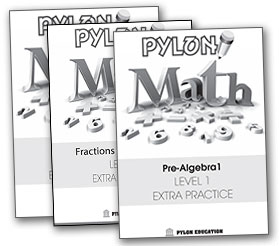Curriculum

Self-Independent Math Workbook provides easy-to-learn methods of math.  We also offer math tutoring options.

Algebra / Geometry WorkbooksPreAlgebra 1

6th / 7th Grade - Click for details

• Variable Expressions
• Order of Operations
• Basic Properties and Equations
• Prime and Composite Numbers
• Capacity
• Ratios
• Percent Proportions
• Percent EquationsPreAlgebra 2

7th / 8th Grade - Click for details

• Equations
• Word Problems
• Percents
• Interest
• The Coordinate Planes
• Box-and-Whisker Plots
• Probability
• Mixed Review
• Areas
• Circles
• Volumes
• Surface Areas
• Special Right Triangles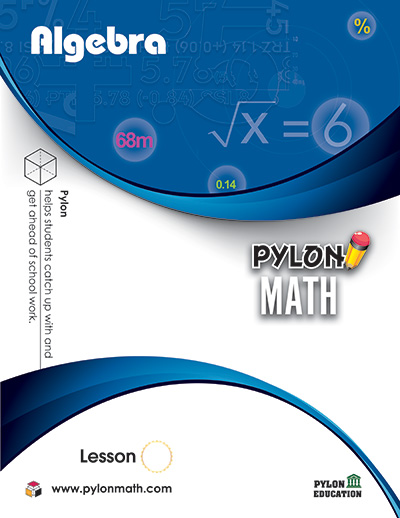Algebra

8th / 9th Grade - Click for details

• Equations
• Word Problems
• Percent Word Problems
• The Coordinate Planes
• Systems of Equations
• Inequalities
• Polynomials
• Factoring
• Rational Expressions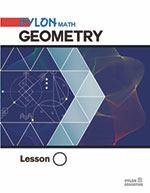Geometry

9th / 10th Grade - Click for details

• Proofs
• Parallel Lines
• Congruent Triangles
• Angles
• Parallelograms
• Transformations
• Circles
• Right Triangles and Trigonometry
• Area of Polygons and Circles
• Surface Area and VolumeAlgebra 2

10th / 11th Grade - Click for details

• Absolute Value Inequality
• Functions
• Graphs
• Matrices
• Polynomials
• Logarithmic Functions
• Rational Functions
• Irrational Numbers
• Conic Sections
• Sequences
• Statistics

Math Word Problem WorkbooksWord Problem Level C

3rd Grade - Click for details

• Place Values
• Subtraction
• Money & Time
• Multiplication
• Multiplication & Patterns
• Division
• Graphs
• Customary Units
• Geometric Figures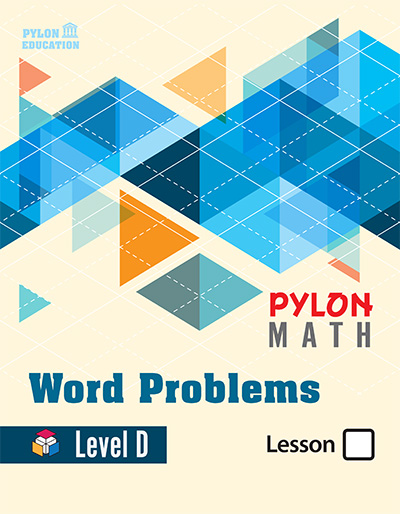Word Problem Level D

4th Grade - Click for details

• Place Values
• Sum & Difference
• Expression and Elapsed Time
• Graphs and Properties
• Multiplication
• Division
• Divisibility Rules
• Geometric Figures
• Metric Units and Weights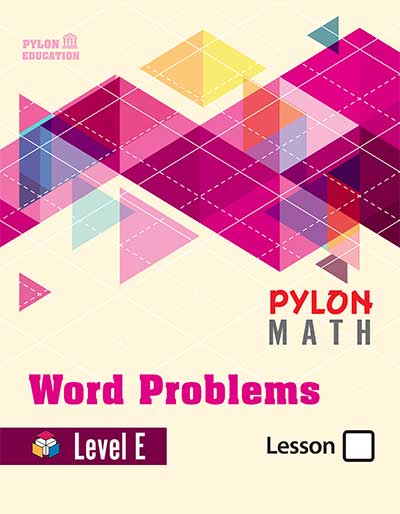Word Problem Level E

5th Grade - Click for details

• Place Values
• Fractions
• Subtracting Fractions
• Multiplying Fractions
• Dividing Fractions
• Decimals
• Dividing Decimals
• Geometry and Other Topics
• Graphs